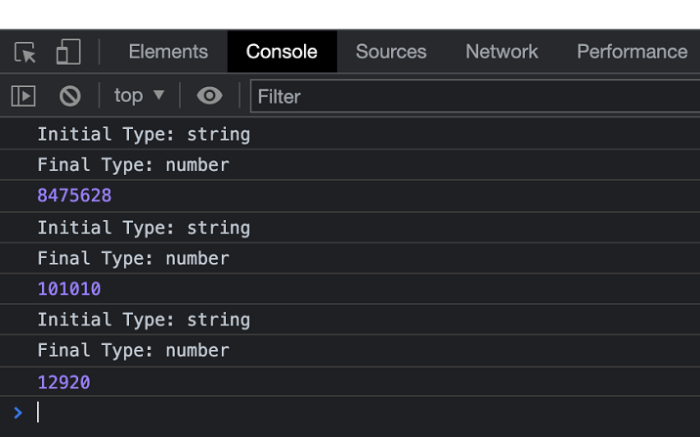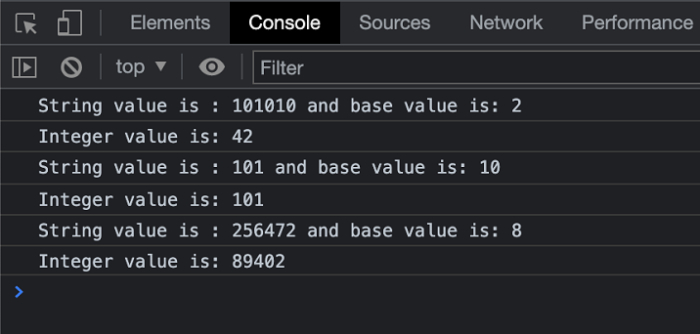# How to convert a string of any base to an integer in JavaScript?

An integer can be represented in various formats as described in the computed languages i.e. Binary, Decimal, Hexadecimal, etc. A Binary number consists of two digits only i.e. 0 & 1, whereas a decimal consists of digits from 0 to 9.

We can convert a string to an integer by using the parseInt() conversion by default. But in other cases when the integer is represented in some other form we also need to pass the base string to decode it into the resultant number.

Below is the syntax of parsing a string to an int.

## Syntax

1. When the base value is provided

parseInt(string_value, base)

2. When the base value is not provided

parseInt(string_value)

By default, the parseInt() method converts into a decimal value.

## Example 1

In the below example, we are converting a string value into an Integer by using the default method and without passing the base value.

# index.html

<!DOCTYPE html>
<html>
<title>
Creating Objects from Prototype
</title>
<body>
<h2 style="color:green">
Welcome To Tutorials Point
</h2>
</body>
<script>
let stringConversion = (string_value) => {
console.log("Initial Type: " + typeof string_value);
let integer_value = parseInt(string_value);
console.log("Final Type: " + typeof integer_value);
console.log(integer_value);
};
stringConversion("8475628");
stringConversion("101010");
stringConversion("0x3278");
</script>
</html>

## Output

On successful execution of the above program, the browser will display the following result −

Welcome To Tutorials Point

And in the console, you will find all the results, see the screenshot below −## Example 2

In the below example, we are converting a string value into an Integer by also passing the base value along with the string.

# index.html

<!DOCTYPE html>
<html>
<title>
Creating Objects from Prototype
</title>
<body>
<h2 style="color:green">
Welcome To Tutorials Point
</h2>
</body>
<script>
let StringConversion = (string_value, base) => {
console.log(
"String value is : " + string_value +
" and base value is: " + base
);
let Integer_value = parseInt(string_value, base);
console.log("Integer value is: " + Integer_value);
};
StringConversion("101010", 2);
StringConversion("101", 10);
StringConversion("256472", 8);
</script>
</html>

## Output

On successful execution of the above program, the browser will display the following result −

Welcome To Tutorials Point

And in the console, you will find all the results, see the screenshot below −